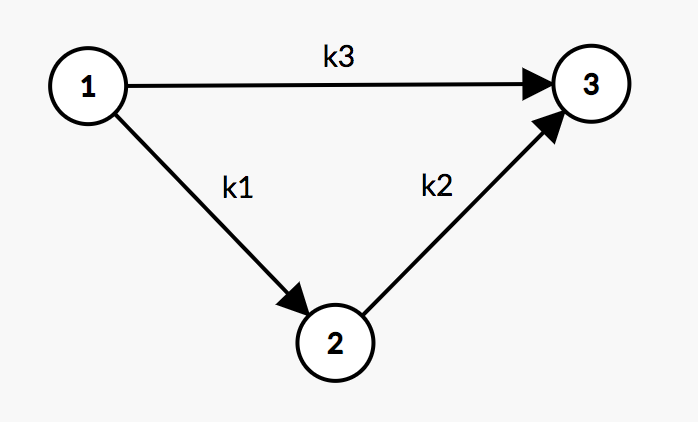# POJ3169 Layout（差分约束+最短路）

## Layout

Like everyone else, cows like to stand close to their friends when queuing for feed. FJ has N (2 <= N <= 1,000) cows numbered 1..N standing along a straight line waiting for feed. The cows are standing in the same order as they are numbered, and since they can be rather pushy, it is possible that two or more cows can line up at exactly the same location (that is, if we think of each cow as being located at some coordinate on a number line, then it is possible for two or more cows to share the same coordinate).

Some cows like each other and want to be within a certain distance of each other in line. Some really dislike each other and want to be separated by at least a certain distance. A list of ML (1 <= ML <= 10,000) constraints describes which cows like each other and the maximum distance by which they may be separated; a subsequent list of MD constraints (1 <= MD <= 10,000) tells which cows dislike each other and the minimum distance by which they must be separated.

Your job is to compute, if possible, the maximum possible distance between cow 1 and cow N that satisfies the distance constraints.

### Input

Line 1: Three space-separated integers: N, ML, and MD.

Lines 2..ML+1: Each line contains three space-separated positive integers: A, B, and D, with 1 <= A < B <= N. Cows A and B must be at most D (1 <= D <= 1,000,000) apart.

Lines ML+2..ML+MD+1: Each line contains three space-separated positive integers: A, B, and D, with 1 <= A < B <= N. Cows A and B must be at least D (1 <= D <= 1,000,000) apart.

### Output

Line 1: A single integer. If no line-up is possible, output -1. If cows 1 and N can be arbitrarily far apart, output -2. Otherwise output the greatest possible distance between cows 1 and N.

### Sample Input

4 2 1
1 3 10
2 4 20
2 3 3

### Sample Output

27

### Hint

Explanation of the sample:

There are 4 cows. Cows #1 and #3 must be no more than 10 units apart, cows #2 and #4 must be no more than 20 units apart, and cows #2 and #3 dislike each other and must be no fewer than 3 units apart.

The best layout, in terms of coordinates on a number line, is to put cow #1 at 0, cow #2 at 7, cow #3 at 10, and cow #4 at 27.

### 链接

https://cn.vjudge.net/problem/POJ-3169【为什么是vjudge的链接呢，因为POJ挂了】

### 题意

n头奶牛，标号为1到n，排成一列，先给定其中一些奶牛的关系：

• ml，奶牛A和奶牛B的距离小于等于D
• md，奶牛A和奶牛B的距离大于等于D

### 代码

• 未提交POJ、暂挂
#include <iostream>
#include <cstdio>
#include <cstring>
#include <algorithm>
#include <queue>
#include <vector>
using namespace std;
const int inf = 0x3f3f3f3f;
const int maxn = 1010;

struct Edge
{
int from, to;
int dist;
Edge(int _from, int _to, int _dist): from(_from), to(_to), dist(_dist) {}
};

struct BellmanFord // 最短路
{
int n, m;
vector <Edge> edges;
vector <int> G[maxn];
bool inq[maxn];
int d[maxn];
int p[maxn];
int cnt[maxn];

void init(int n)
{
this->n = n;
for (int i = 0; i < n; ++i)
G[i].clear();
edges.clear();
}

void addedge(int from, int to, int dist)
{
edges.push_back(Edge(from, to, dist));
m = edges.size();
G[from].push_back(m - 1);
}

bool negativeCycle()
{
queue <int> Q;
memset(inq, 0, sizeof(inq));
memset(cnt, 0, sizeof(cnt));
for (int i = 0; i < n; ++i)
d[i] = inf;
d = 0;
inq = true;
Q.push(0);
while (!Q.empty())
{
int u = Q.front();
Q.pop();
inq[u] = false;
for (int i = 0; i < G[u].size(); ++i)
{
Edge & e = edges[G[u][i]];
if (d[e.to] > d[u] + e.dist)
{
d[e.to] = d[u] + e.dist;
p[e.to] = G[u][i];
if (!inq[e.to])
{
Q.push(e.to);
inq[e.to] = true;
if (++cnt[e.to] > n)
return true;
}
}
}
}
return false;
}
};

int main()
{
//    freopen("/Users/taifu/Codes/NOW/data.in", "r", stdin);
//    freopen("/Users/taifu/Codes/NOW/data.out","w",stdout);
int n, ml, md, u, v, w;
BellmanFord bm;
while (scanf("%d%d%d", &n, &ml, &md) != EOF)
{
bm.init(n);
for (int i = 0; i < ml; ++i)
{
scanf("%d%d%d", &u, &v, &w);
u--, v--;
bm.addedge(u, v, w); // u v w
}
for (int i = 0; i < md; ++i)
{
scanf("%d%d%d", &u, &v, &w);
u--, v--;
bm.addedge(v, u, -w); // v u -w
}
bool flag = bm.negativeCycle();
if (!flag)
{
if (bm.d[n-1] == inf) // 距离无限长
printf("-2\n");
else
printf("%d\n", bm.d[n-1]); // 答案
}
else // 存在负权回路
printf("-1\n");
}
return 0;
}

### 详解（转载自传送门）

1、因为我们要求1号牛和n号牛的最大距离，那么我们希望得到这样一个不等式(假设n==5)：①-⑤<=x，那么这个x就是1号牛和n号牛的最大距离。

2、那么我们引入一个差分约束系统的相关概念：

$x_1 - x_2 <= k_1$

$x_2 - x_3 <= k_2$

$x_1 - x_3 <= k_3$

$x_1 - x_3 <= k_1 + k_2$

$x_1 - x_3 <= k_3$3、然后我们再回归到这个问题上来。

4、图建立好之后直接跑最短路即可。

①如果dis【n】==inf，输出-2；

②否则输出dis【n】；

③如果在跑贝尔曼福德过程中发现了负环，说明问题无解，那么输出-1.

The end.
2018-09-05 星期三